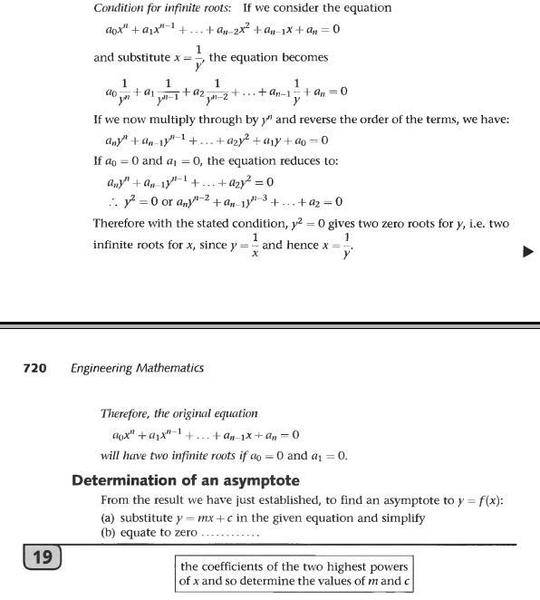# Is this condition for infinite roots wrong?

• I
• vopros217

#### vopros217

I found a strange theorem and a doubtful method in Stroud's book "Engineering mathematics":I think, every polynomial equation will have two infinite roots (at +infinity and -infinity).
I also think that this method of the determination of an asymptote gives wrong results if f(x) is a polynomial with a high degree.
Are the theorem and the method in this book wrong?

Last edited:
That doesn't make sense. Every polynomial has a largest power, so every polynomial has an infinite number of infinite roots?
Multiplying the equation by yn generates additional solutions for y=0, so it is not surprising that they produce unmathematical solutions for y=0 later. Of course y=0 is a solution to an equation where you multiplied both sides by 0.

•vopros217
This looks strange but it is kind of correct. It seems the author absolutely butchered the method though. I think what the author likes to do is to compute infinite point and asymptotes in projective geometry using homogeneous coordinates. That uses a method kind of similar to what is in the OP, but the exact explanation of the book is very doubtful.

Something of the following would be correct though: consider ##y = \frac{x+2}{3x + 2}##. Then we have
$$3xy + 2y - x - 2 = 0$$
which gives rise to a homogeneous equation
$$3xy + 2yz - xz - 2z^2=0.$$
The points at infinity correspond to ##z=0##, which yields ##x=0## or ##y=0##. This gives us a result that the function has two asymptotes: one parallel to ##x=0## and one parallel to ##y=0## which is indeed correct.

•vopros217
Statement is strange. if $a_0\ and\ a_1$ are both 0, then the first term should be $a_2x^{n-2}$.

•vopros217
Thank you all. Stroud's textbook is mostly very good, but I stuck on that place.